Next: Non-Interacting Particles Up: Multi-Particle Systems Previous: Introduction

# Fundamental Concepts

We have already seen that the instantaneous state of a system consisting of a single non-relativistic particle, whose position coordinate is, is fully specified by a complex wavefunction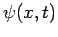. This wavefunction is interpreted as follows. The probability of finding the particle betweenand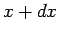at timeis given by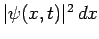. This interpretation only makes sense if the wavefunction is normalized such that(417)

at all times. The physical significance of this normalization requirement is that the probability of the particle being found anywhere on the-axis must always be unity (which corresponds to certainty).

Consider a system containingnon-relativistic particles, labeled, moving in one dimension. Letandbe the position coordinate and mass, respectively, of the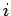th particle. By analogy with the single-particle case, the instantaneous state of a multi-particle system is specified by a complex wavefunction. The probability of finding the first particle between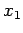and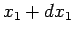, the second particle between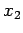and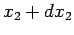, etc., at timeis given by. It follows that the wavefunction must satisfy the normalization condition(418)

at all times, where the integration is taken over all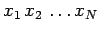space.

In a single-particle system, position is represented by the algebraic operator, whereas momentum is represented by the differential operator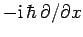(see Sect. 4.6). By analogy, in a multi-particle system, the position of theth particle is represented by the algebraic operator, whereas the corresponding momentum is represented by the differential operator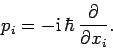(419)

Since theare independent variables (i.e.,), we conclude that the various position and momentum operators satisfy the following commutation relations: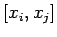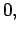(420)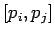(421)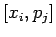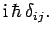(422)

Now, we know, from Sect. 4.10, that two dynamical variables can only be (exactly) measured simultaneously if the operators which represent them in quantum mechanics commute with one another. Thus, it is clear, from the above commutation relations, that the only restriction on measurement in a one-dimensional multi-particle system is that it is impossible to simultaneously measure the position and momentum of the same particle. Note, in particular, that a knowledge of the position or momentum of a given particle does not in any way preclude a similar knowledge for a different particle. The commutation relations (420)-(422) illustrate an important point in quantum mechanics: namely, that operators corresponding to different degrees of freedom of a dynamical system tend to commute with one another. In this case, the different degrees of freedom correspond to the different motions of the various particles making up the system.

Finally, if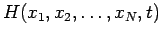is the Hamiltonian of the system then the multi-particle wavefunctionsatisfies the usual time-dependent Schrödinger equation [see Eq. (199)]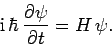(423)

Likewise, a multi-particle state of definite energy(i.e., an eigenstate of the Hamiltonian with eigenvalue) is written (see Sect. 4.12)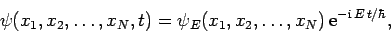(424)

where the stationary wavefunction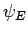satisfies the time-independent Schrödinger equation [see Eq. (296)]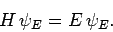(425)

Here,is assumed not to be an explicit function of.Next: Non-Interacting Particles Up: Multi-Particle Systems Previous: Introduction
Richard Fitzpatrick 2010-07-20